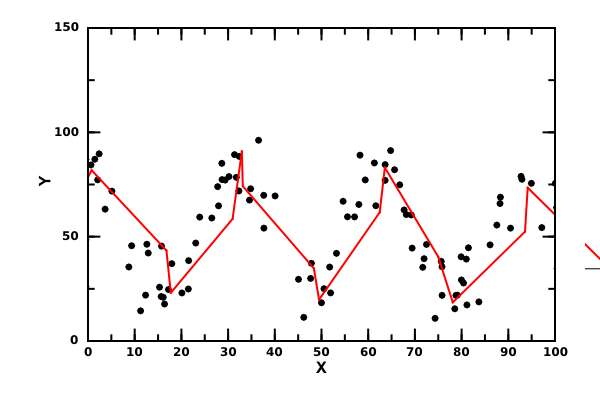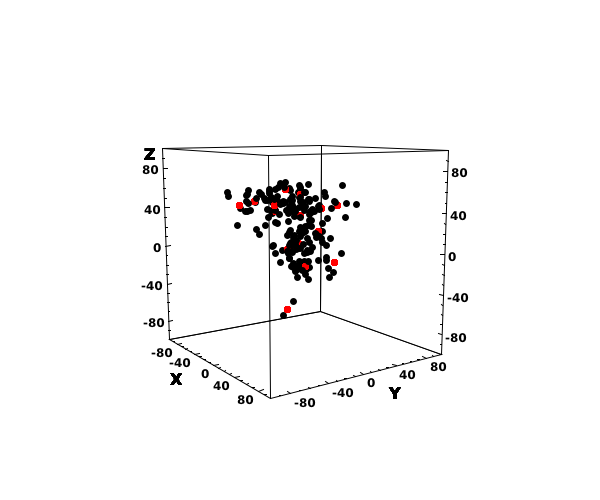# DMelt:AI/3 Kohonen Maps

Limitted access. First login to DataMelt if you are a full DataMelt member. Then login to HandWiki as a user.

# Kohonen SOM

Kohonen Self-Organizing Maps (or just Self-Organizing Maps, or SOM for short) is a type of artificial neural network that is trained using unsupervised learning to produce a two-dimensional discretized representation of the input space of the training samples, called a map.

These maps are useful for classification and visualizing low-dimensional views of high-dimensional data, akin to multidimensional scaling. The model was first described as an artificial neural network by the Finnish professor Teuvo Kohonen, and is sometimes called a Kohonen Map. See Self-organizing_map article.

The SOM may be described as a nonlinear, ordered, smooth mapping of high-dimensional input data onto the elements of a regular, low-dimensional array. In its basic form it produces a similarity graph of input data.

The SOM converts the nonlinear statistical relationships between high-dimensional data into simple geometric relationship of their image points on a regular two-dimensional grid of nodes. The SOM maps can be used for classification and visualizing of high-dimensional data.

1.2. Learning Algorithm

Unlike many other types of neural nets, the SOM doesn't need a target output to be specified. Instead, where the node weights match the input vector, that area of the lattice is selectively optimized to more closely resemble the data for the class the input vector is a member of.

# Training

From an initial distribution of random weights, and over many iterations, the SOM eventually settles into a map of stable zones. Each zone is effectively a feature classifier, so you can think of the graphical output as a type of feature map of the input space.

Training occurs in several steps and over many iterations:

1. Each node's weights are initialized with random values.
2. A vector is chosen randomly from the set of training data.
3. Every node is examined to calculate which one's weights are most like the input vector. The winning node is commonly known as the Best Matching Unit (BMU).
4. The radius of the neighborhood of the BMU is calculated. Initially, this value is set to the radius of the lattice, but diminish each time step.
5. For any nodes found inside the radius of BMU, the node's weights are adjusted to make them more like the input vector. The closer a node to the BMU, the more its weights get alerted.
6. Repeat step 2 for N iterations.

Below we will consider a number of examples of how to construct and run Kohonen Self-Organizing Maps. The code is implemented in Java language. But, as before, we will use Python scripting to make the SOM examples shorter. The Kohonen SOM examples will be based on the Java classjhpro.nnet.KohonenFeatureMap. We should acknowledge the work of Jochen Fröhlich who has create a first version of this Java class.

# Kohonen SOM in 2D

Now we will consider a Kohonen Self-Organizing Map in 2D space. In this example we generate random numbers in X-Y space and then apply the Kohonen Self-Organizing Map algorithm with 4x4 neurons. Then we perform learning and update the plot every 50 iterations, until the area is equal to 0.01.

Note that we shod weights as lines. Therefore, to make a visually appealing plot, we sort the weight array to draw lines. You can remove the line option and then the sort() function will not be needed.

The resulting answer is shown below. Note that this image shows the final result after the learning stop.# Kohonen SOM in 3D

Now we will consider a Kohonen Self-Organizing Maps network in 3D space. This Python example is very similar to the 2D case. Only now we show the resulting weights as red dots in the 3D space:

The result of the above example is shown below:# Self-Organizing Maps in Python

Another example of the Python coding is a Self-Organizing Maps implemented in a self-contained Python code:

Look at the online examples ("Tools"-"Online examples"): Artificial Intelligence/neural net/neural_net_som.py Open it and run by pressing [F8].